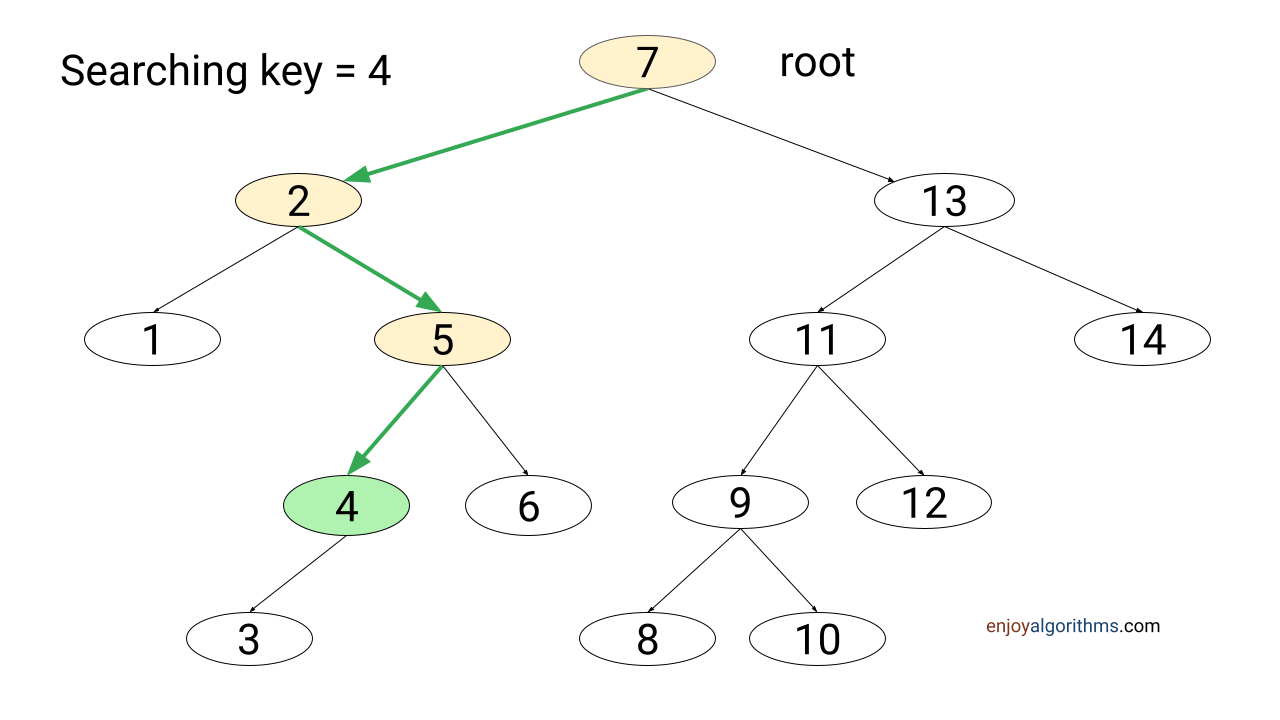# Search Operation in Binary Search Tree

Problem statement: The root of a binary search tree and key k is given in the input. Write a program to search key k into the binary tree. If key k is present, return a pointer to the node containing key k. Otherwise, return NULL.

### Searching in a BST using recursion

#### Solution idea

A binary search tree is a sorted binary tree, where the root->key is greater than the keys of all nodes in the left subtree and less than the keys of all nodes in the right subtree. So we can apply the divide and conquer idea similar to binary search, i.e. we compare root->key with k and divide the searching problem into one smaller sub-problem of searching either left or right sub-tree.

As we go down searching in a BST, the size of search space or subtree rooted at the current node reduces max by half (when the tree is balanced) but at least by one (when the tree is skewed). The search process stops either when a node containing the search key is found (search hit) or we reach one of the NULL links (search miss).#### Solution steps

As discussed above, the recursive algorithm to search for a key in a BST follows a simple recursive structure: if key k is equal to the root->key, we have a search hit. Otherwise, we search recursively in the appropriate subtree.

Suppose we define a function BSTNode recursiveBSTSearch(BSTNode root, int k), which takes root and search key k as an input.

• If (root->key == k), we return root.
• If (root->key > k): All keys in the right subtree are greater than root->key, so we search recursively in the left subtree by calling the same function for root->left, i.e. recursiveBSTSearch(root->left, k).
• If (root->key < k): All keys in the left subtree are less than root->key, so we search recursively in the right subtree by calling the same function for root->right, i.e. recursiveBSTSearch(root->right, k).

#### Solution pseudocode

``````BSTNode recursiveBSTSearch(BSTNode root, int k)
{
if (root == NULL || root->key == k)
return root

if (root->key > k)
return bstSearch(root->left, k)
else
return bstSearch(root->right, k)
}``````

### Searching in BST using iteration

#### Solution idea

We can iteratively rewrite the above procedure by unrolling the recursion into a while loop. We start searching from the root node and traverse a path iteratively downward in the tree. For each node x in the path, we compare k with x->key. If both are equal, the search is successful. If k is smaller than the x->key, we search iteratively in the left subtree of x. Similarly, if k is larger than the x->key, we search iteratively in the right subtree.

#### Solution steps

We run a while loop and perform the following operations. Loop will run till root->key != k or root != NULL.

• If root->key > k, we set root = root->left.
• If root->key < k, we set root = root->right.
• If the loop exits due to root == NULL, there is a search miss.
• If the loop exit due to root->key == k, there is a search hit.
``````BSTNode iterativeBSTSearch(BSTNode root, int k)
{
while (root != NULL && root->key != k)
{
if (root->key > k)
root = root->left
else
root = root->right
}
return root
}``````

### Time complexity analysis

Nodes encountered during the recursion or iteration generate a path from the root node. If the search is successful, the path terminates at the node containing the key. Otherwise, it will traverse a path from the root node to one of the NULL links.

So search method will perform one comparison at each level of the tree. In the worst case, the total number of comparison operations equals the tree's height (h). The time complexity of searching in BST = The height of BST * O(1) = h * O(1) = O(h).

When BST is balanced, both the left and right subtree will be the same size (n/2), and the height of BST will be O(logn). So time complexity of searching in a balanced BST = O(logn)

When BST is completely unbalanced, the BST will be a linear chain of nodes (one node at each level), and the height of such BST is O(n). So time complexity of searching in an unbalanced BST = O(n)

### Space complexity analysis

We use constant extra memory in iterative implementation, so the space complexity of iterative search operation in BST = O(1). There will be one recursive call at each tree level in the recursive implementation. So space complexity depends on the size of the recursion call stack, which is equal to the tree's height. so space complexity of recursive search operation in BST = O(h)

• For balanced BST, space complexity = O(logn).
• For a completely unbalanced BST, space complexity = O(n).

Enjoy learning, enjoy algorithms!

☆ 16-Week Live DSA Course
☆ 10-Week Live DSA Course